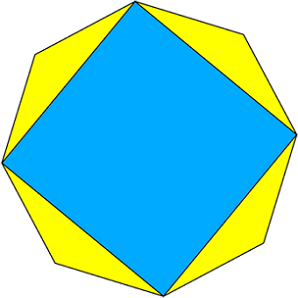# Dissecting A Square

Geometry Level 2Given a regular octagon (8 sides), I connect each alternating vertice to form a square. If the area of this square is $2+\sqrt2$, what is the perimeter of the octagon?

×